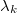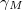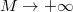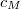﻿

### Ingham type inequalities towards Parseval equality

#### Abstract

We consider Trigonometric series with real exponents$\lambda_k$:
Under an assumption on the gap$\gamma_M$ between$\lambda_k$, we show the inequality \begin{equation*}\label{conf000} \frac {2\pi}{\gamma_M(2-c_M)}\sum_{n=1}^M\vert x_n\vert^2 \leq \int_{-\pi/\gamma_M}^{\pi/\gamma_M}\vert \sum_{k=1}^{M} x_ke^{i \lambda_kt}\vert^2dt\leq \frac {2\pi}{c_M\gamma_M} \sum_{n=1}^M\vert x_n\vert^2 \end{equation*} and we show for a class of problems that the limit as$M\to + \infty$ leads to the Parseval's equality. The role of constants$c_M$ in the above formula is one of the key points of the paper

Keywords: Trigonometric polynomials; inequalities; Parseval equality

Full Text: PDF